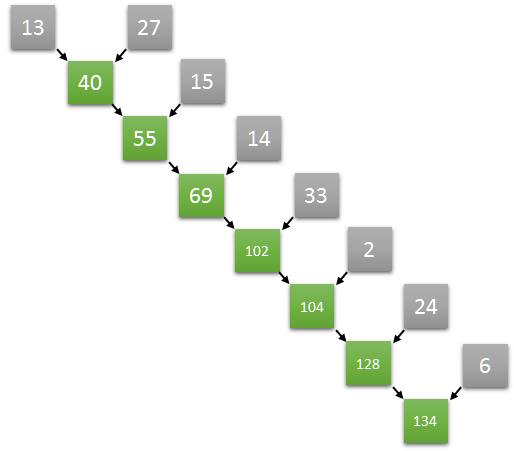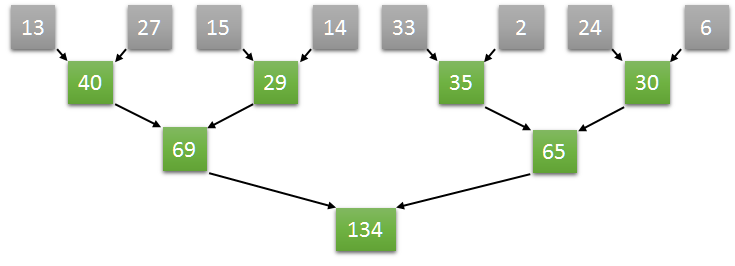Reduction operations are those that reduce a collection of values to a single value. In this post, I will share how to implement parallel reduction operations using CUDA.

## Sequential Sum

Compute the sum of all elements of an array is an excellent example of reduction operation. The sum of an array which values are 13, 27, 15, 14, 33, 2, 24, and 6 is 134.

The interesting question is: How would you compute it? Probably your first answer would be doing something like this (((((((13+27)+15)+14)+33)+2)+24)+6). Am I right?The problem with this approach is that it is impossible to parallelize. Why? Each step depends on the result of the previous one.

## Parallel Sum

Adding values is an associative operation. So, we can  try something like this ((13+27)+(15+14))+((33+2)+(24+6))This way is much better because now we can execute it in parallel!

## CUDA

Let’s figure out how to do it using CUDA.Here is the main idea:

• Assuming N as the number of the elements in an array, we start N/2 threads, one thread for each two elements
• Each thread computes the sum of the corresponding two elements, storing the result at the position of the first one.
• Iteratively, each step:
• the number of threads halved (for example, starting with 4, then 2, then 1)
• doubles the step size between the corresponding two elements (starting with 1, then 2, then 4)
• after some iterations, the reduction result will be stored in the first element of the array.

Let’s code

```#include "cuda_runtime.h"
#include "device_launch_parameters.h"

#include <iostream>
#include <numeric>
using namespace std;

__global__ void sum(int* input)
{

auto step_size = 1;

{
if (tid < number_of_threads) // still alive?
{
const auto fst = tid * step_size * 2;
const auto snd = fst + step_size;
input[fst] += input[snd];
}

step_size <<= 1;
}
}

int main()
{
const auto count = 8;
const int size = count * sizeof(int);
int h[] = {13, 27, 15, 14, 33, 2, 24, 6};

int* d;

cudaMalloc(&d, size);
cudaMemcpy(d, h, size, cudaMemcpyHostToDevice);

sum <<<1, count / 2 >>>(d);

int result;
cudaMemcpy(&result, d, sizeof(int), cudaMemcpyDeviceToHost);

cout << "Sum is " << result << endl;

getchar();

cudaFree(d);
delete[] h;

return 0;
}
```

## Time to action

In this post, we implemented a primary example of parallel reduction operation in CUDA. But, the pattern we adopted can be used in more sophisticated scenarios.

I strongly recommend you to try to implement other reduction operations (like discovering the max/min values of an array). Now, it would be easy. Right?

### This Post Has 6 Comments

1.Luís Barbosa

Hi Elemar,

what do you think about projects like Hybridizer and ManagedCuda?

1.elemarjr

I’ve been testing ALEA.. It is “nice”

2.Shivani Shinde

What do following lines do?
step_size <>= 1;
Or partiularly, what is functioning of “<>==”?

1.Rahul

The line is:
step_size<<=1
It doubles the step_size.

3.Liangzhi Tang

How to sum using multiple blocks?

4.me

hi, what happens when the size of the array is not a power of two? Im trying to do it with an array of 784 elemnts but it does no sum well, do you have any ideas? thanks in advance